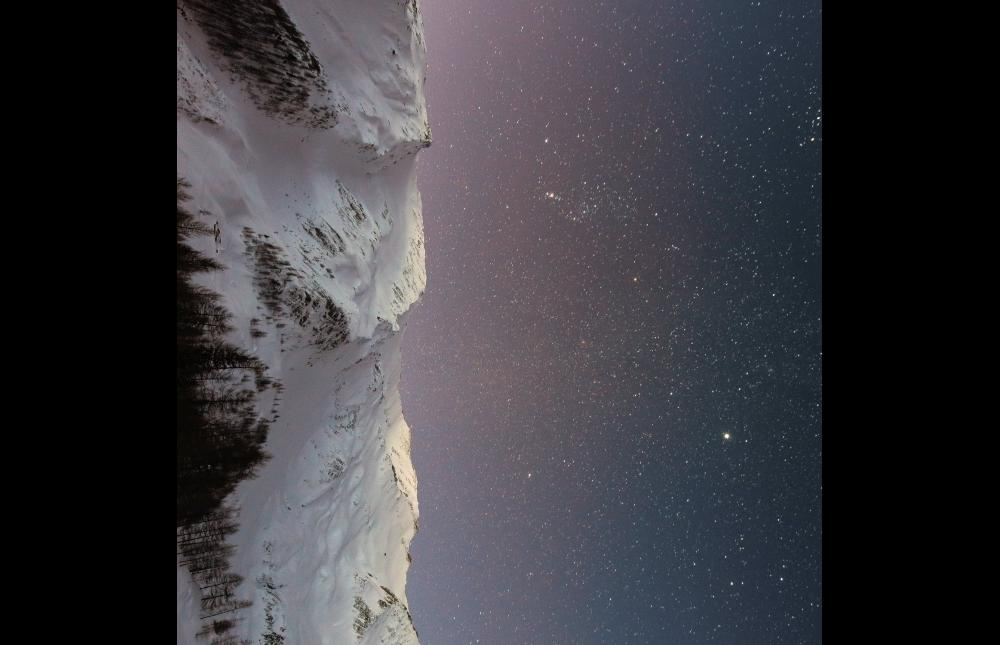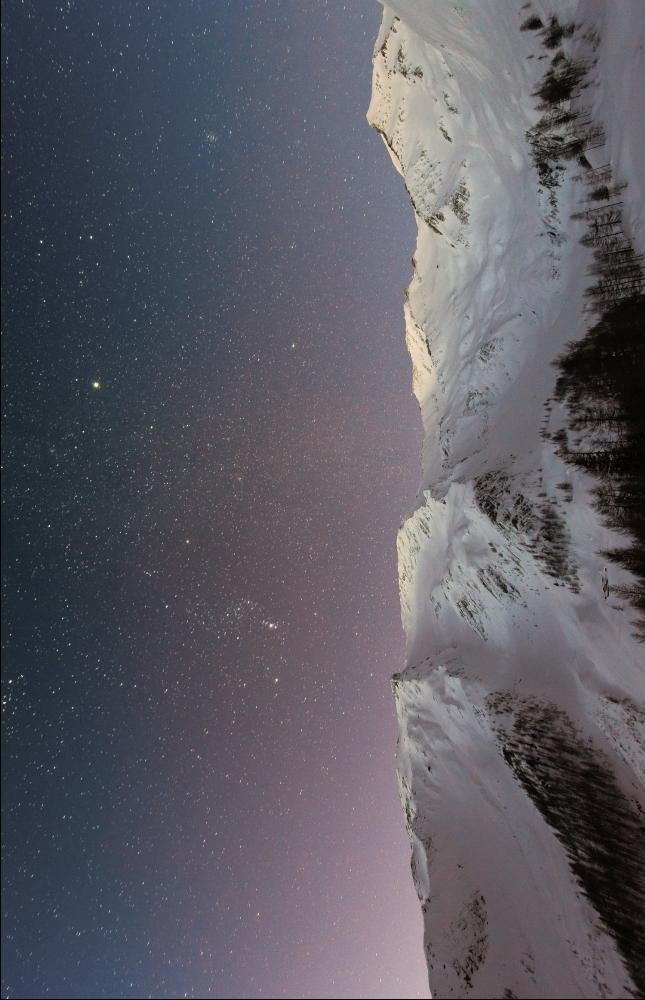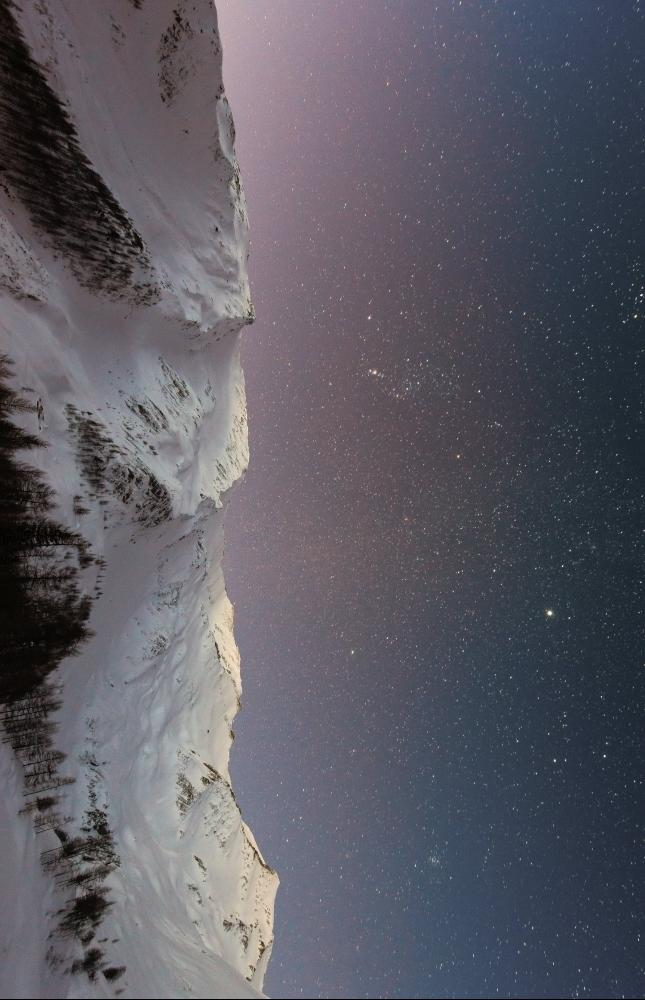# Rotate an Image in Java

Rupam Yadav Jan 30, 2023 Sep 06, 2021

This article will introduce how we can rotate an image in Java using two native ways.

## Rotate an Image in Java Using `BufferedImage` and `Graphics2D.rotate()`

The first method to rotate an image includes the use of the `BufferedImage` and the `Graphics2d` class that comes with the AWT package. Below we create a function `rotateImage()` that receives a `BufferedImage` object as a parameter and returns a rotated `BufferedImage` object.

In `rotateImage()`, we get the width, height and the type of the image using `getWidth()`, `getHeight()` and `getType()` methods. Now we call the `BufferedImage()` constructor and pass the three variables as arguments and it returns a `BufferedImage` object `newImageFromBuffer`.

We create new rotated image using `createGraphics()` method that returns a `Graphics2D` object `graphics2D`. Using this object, we call the `rotate()` function that takes three arguments; the first is the angle to rotate the image as we want to rotate it 90 degrees we pass `Math.radians(90)`, the second and third arguments are the x and y coordinates.

Finally, we call `graphics2D.drawImage()` to draw the rotated image, which takes four arguments, the `BufferedImage` object, the filter to apply, and the x and y coordinates. Then we return the `newImageFromBuffer` object.

In the `main` function, we read the file using `File` and then convert it into a `BufferedImage` object using `ImageIO.read()`. Now we call the `rotateImage()` function and pass the returned `BufferedImage` object and store the rotated `BufferedImage` object. Now that we have the rotated image, we need to create a new file to store it using `File` and `ImageIO.write()` that takes the `BufferedImage` object, its extension the location of the empty file.

``````import javax.imageio.ImageIO;
import java.awt.*;
import java.awt.image.BufferedImage;
import java.io.File;
import java.io.IOException;

public class RotateImage {

public static BufferedImage rotateImage(BufferedImage imageToRotate) {
int widthOfImage = imageToRotate.getWidth();
int heightOfImage = imageToRotate.getHeight();
int typeOfImage = imageToRotate.getType();

BufferedImage newImageFromBuffer = new BufferedImage(widthOfImage, heightOfImage, typeOfImage);

Graphics2D graphics2D = newImageFromBuffer.createGraphics();

graphics2D.rotate(Math.toRadians(90), widthOfImage / 2, heightOfImage / 2);
graphics2D.drawImage(imageToRotate, null, 0, 0);

return newImageFromBuffer;
}

public static void main(String[] args) {

try {

BufferedImage subImage = rotateImage(originalImage);

File rotatedImageFile = new File("C:\\Users\\User1\\Documents\\mountainsRotated.jpeg");

ImageIO.write(subImage, "jpg", rotatedImageFile);

System.out.println("New Rotated Image File Path: "+rotatedImageFile.getPath());

} catch (IOException e) {
e.printStackTrace();
}
}

}
``````

Output:

``````New Rotated Image File Path: C:\Users\User1\Documents\mountainsRotated.jpeg
``````

Original Image:Rotated Image:## Rotate an Image in Java Using Affine Transform

This example uses the `AffineTransform` class that maps an image from its original 2D coordinates to other 2D coordinates linearly without losing the original quality. In the following program, we three methods, one to read and call other functions, the second is to rotate the image clockwise, and the last function rotates the image counterclockwise.

In the `rotateImage()` function, we read the image using `new File()` and convert it to a `BufferedImage` object using `ImageIO.read()`. Then we create another `BufferedImage` object that preserves the properties of the original image and call it `output`. Next we call the `rotateImageClockWise()` method and pass the original `BufferedImage` in it that returns an object of `AffineTransorm` class.

`rotateImageClockWise()` receive the `image` and get the height and width. We create a `AffineTransform` object `affineTransform` and call `rotate()` method using it. In `rotate()`, we pass three arguments; the first is the rotate angle measured in radians, here we pass `Math.PI / 2`, the last two arguments are the x and y coordinates which are half of the width and height of the image.

Now, as the image is rotated, we translate the image in the new coordinates using the `translate()` function that takes two arguments: the distance to rotate in the x-direction and the distance to rotate in the y-direction. We calculate the x and y arguments using `(imageWidth - imageHeight) / 2`.

To rotate the image counter clockwise, we can call the method `rotateImageCounterClockwise()` in `rotate()` instead of `rotateImageClockWise()`.

``````import javax.imageio.ImageIO;
import java.awt.geom.AffineTransform;
import java.awt.image.AffineTransformOp;
import java.awt.image.BufferedImage;
import java.io.File;

public class RotateImage {

private static final String INPUT_FILE_NAME = "mountains.jpeg";
private static final String OUTPUT_FILE_NAME = "mountainsRotated.jpeg";

private AffineTransform rotateImageClockWise(BufferedImage image) {

int imageWidth = image.getWidth();
int imageHeight = image.getHeight();

AffineTransform affineTransform = new AffineTransform();
affineTransform.rotate(Math.PI / 2, imageWidth / 2, imageHeight / 2);

double offset = (imageWidth - imageHeight) / 2;
affineTransform.translate(offset, offset);
return affineTransform;
}

private AffineTransform rotateImageCounterClockwise(BufferedImage image) {

int imageWidth = image.getWidth();
int imageHeight = image.getHeight();

AffineTransform affineTransform = new AffineTransform();
affineTransform.rotate(-Math.PI / 2, imageWidth / 2, imageHeight / 2);

double offset = (imageWidth - imageHeight) / 2;
affineTransform.translate(-offset, -offset);

return affineTransform;
}

private void rotateImage() throws Exception {

BufferedImage output = new BufferedImage(bufferedImage.getHeight(), bufferedImage.getWidth(), bufferedImage.getType());

AffineTransform affineTransform = rotateImageClockWise(bufferedImage);
AffineTransformOp affineTransformOp = new AffineTransformOp(affineTransform, AffineTransformOp.TYPE_BILINEAR);
affineTransformOp.filter(bufferedImage, output);

ImageIO.write(output, "jpg", new File(OUTPUT_FILE_NAME));

}

public static void main(String[] args) {

try {

RotateImage rotateImage = new RotateImage();
rotateImage.rotateImage();

} catch (Exception e) {
e.printStackTrace();
}
}
}
``````

Output:

Original Image:Image Rotated Clockwise:Image Rotated Counter Clockwise: